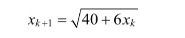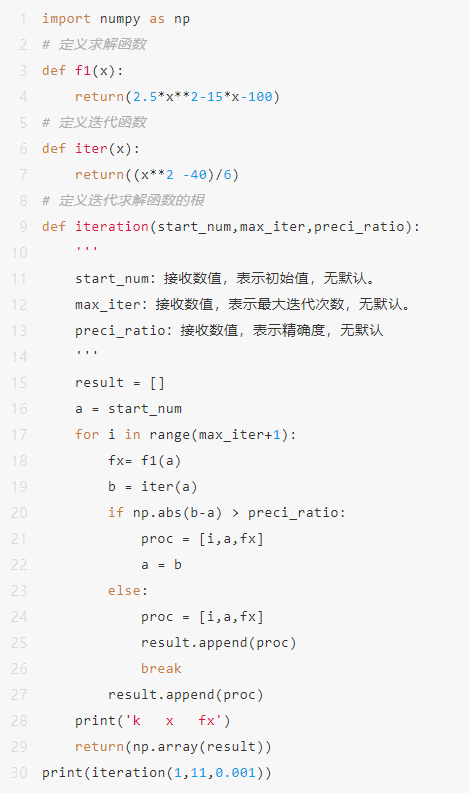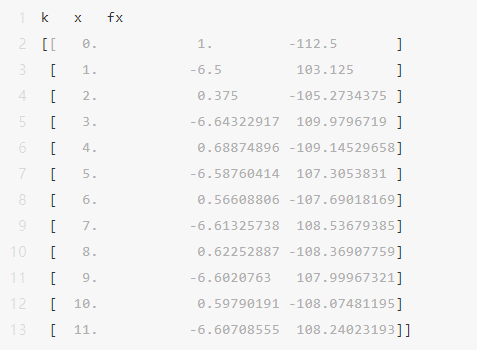2023
05-09

# python数值分析基础教程（python数值计算）scipy.interpolate.lagrange(x,w)# x:接受 array,表示插值节点的x坐标。无默认值# w:接受 array,表示插值节点的y坐标,数目需要与x对应。无默认值假设有一个每年生产240吨产品的食品加工厂，需要统计生产费用，但由于该厂的各项资料不全，无法统计。这种情况下，统计部门收集了设备、生产能力和与该厂大致相同的5个食品加工厂的相关产量和生产费用的资料，如下表所示，请参考数据估计生产费用。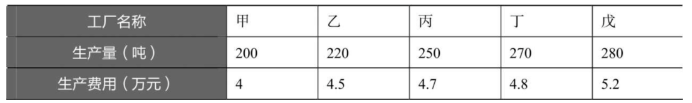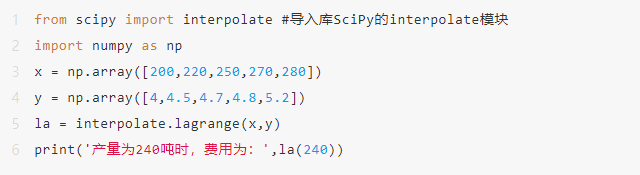1.2牛顿插值法-适合给出插值节点的情况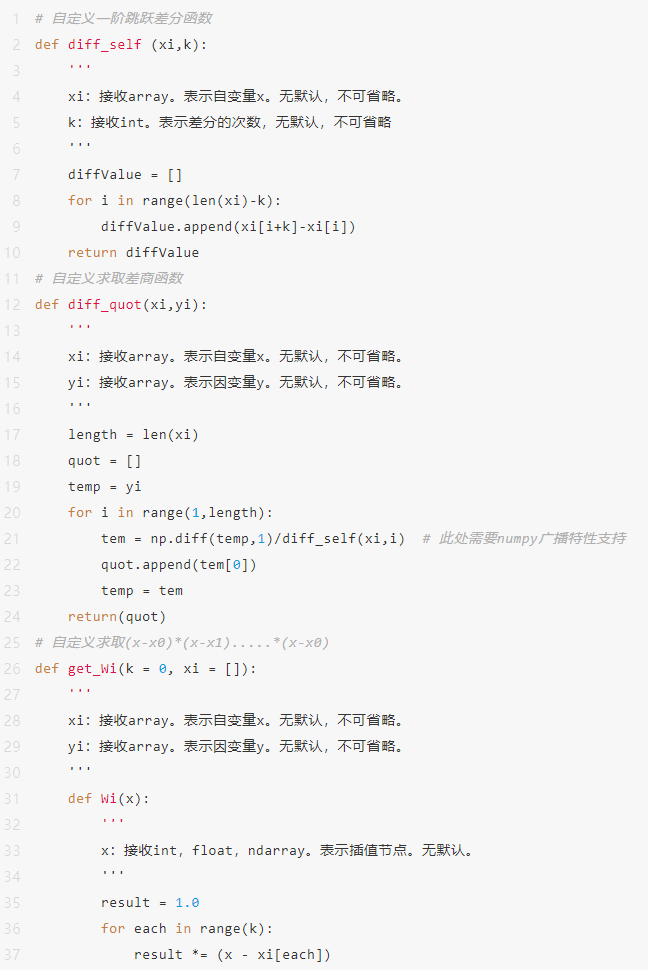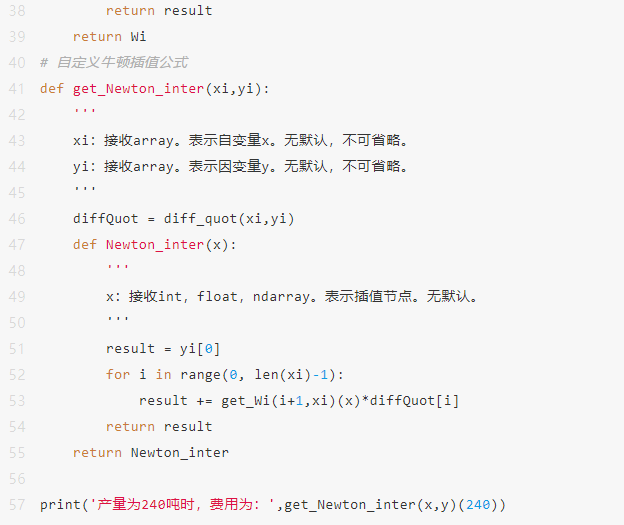SciPy库中的interpolate模块提供了UnivariateSpline类来进行一般的样条插值计算，其语法格式如下。

class scipy.interpolate.UnivariateSpline(x,y,w=None,bbox=[None,None],k=3,s=None,ext=0,check_finite=False)

# x:接受 array,表示插值节点的x坐标。无默认值

# y:接受 array,表示插值节点的y坐标,数目需要与x对应。无默认值

# w:接受 array,表示样条的拟合权重。默认为None

# k:接受 int,表示样条曲线的平滑程度,必须小于或等于5。默认为3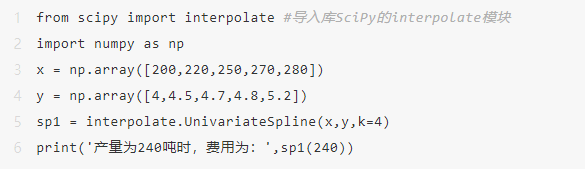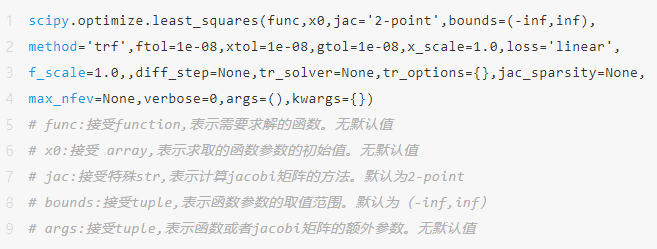import matplotlib.pyplot as plt

from scipy import optimize #导入库SciPy的interpolate模块

import numpy as np

# 拉伸倍数

x = np.array([1.9,2,2.1,2.5,2.7,2.7,3.5,3.5,4,4,4.5,4.6,5,5.2,6,6.3,6.5,7.1,8,8,8.9,9,9.5,10])

# 强度

y = np.array([1.4,1.3,1.8,2.5,2.8,2.5,3,2.7,4,3.5,4.2,3.5,5.5,5,5.5,6.4,6,5.3,6.5,7,8.5,8,8.1,8.1])

plt.rcParams['font.sans-serif']=['SimHei']    # 用来正常显示中文

plt.rcParams['axes.unicode_minus']=False   # 用来正常显示负号

#定义误差

def regula(p):

'''

p:接收tuple、list，表示函数的系数，如ax+b中的a、b。无默认值

'''

a,b = p

return y - a*x-b

# 使用最小二乘法确定a和b

result = optimize.least_squares(regula,[1,0])

a,b = result.x #a,b存储在result的x下

print('线性拟合的结果：a为%s,b为%s'%(a,b))

print('线性拟合的结果展示：')

plt.figure(figsize=(6,4))

plt.scatter(x,y,label='真实值') #绘制原来的点

plt.plot(x,a*x+b,'r',label='拟合直线') #拟合直线

plt.legend()plt.title('纤维线性拟合')

plt.xlabel('拉伸倍数')

plt.ylabel('强度')

plt.xlim(0,11)

plt.ylim(0,9)

plt.savefig('D:/data/纤维线性拟合.png')

plt.show()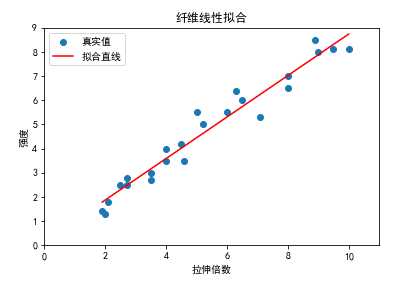（1）求取给定范围内的某个解，而解的粗略位置事先已从问题的物理背景或其他方法得知。

（2）求取方程（组）的全部解，或者求取给定区域内的所有解，而解的个数和位置事先并不知道。这在超越方程的情形下是比较困难的。

3.1、二分法求解非线性方程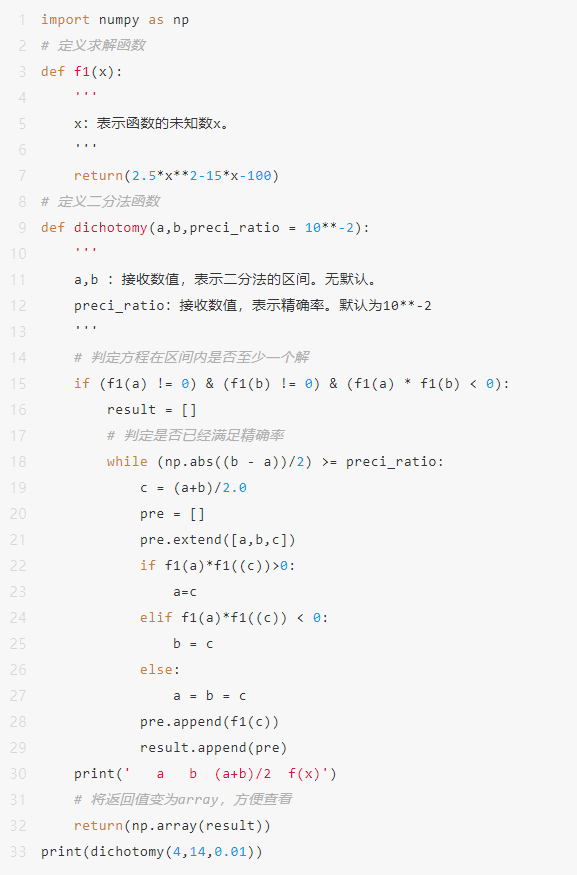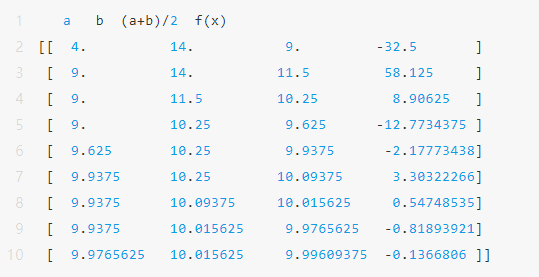3.2、迭代法求解非线性方程